Next: Measurement of Up: Results Previous: Results

### Measurement of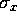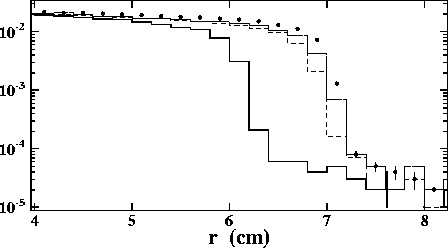Figure 6:   Radial distribution of scattered particles at z=+1m. The solid circles are those of the nominal beam condition; the dotted, dashed and solid lines are those of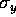=2,=10and=2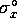, respectively, where the other beam parameters are the same as those of the nominal beam. The distributions are normalized by the total number of particles of E>100MeV.

The maximum radius (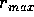) seen in Fig.5(a) corresponds to the maximum deflection angle, since most of the particles obtain transverse momenta mainly due to deflection. Therefore, from a measurement ofwe can obtain information concerning(see Eq.14). A simulation has confirmed thatdepends mainly on, at least for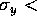10, as shown in Fig.6, wherecan be defined as the shoulder;=7.1 and 6.1cm for the nominal and the case of twice wider, respectively. Small tails due to scattering with large inherent angles are also observed beyond the shoulders. The ratio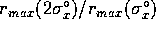is found to be 0.86, which should be compared with 0.88 estimated by Eq.14. In order to estimatefrom the observedwe have to know the beam intensity (N); it can be accurately measured by using monitors in the accelerators. We therefore expect a very good on-line measurement of.

Toshiaki Tauchi
Sat Dec 21 00:34:16 JST 1996